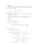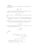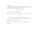# Exercise sheet 3 pdfLoading previews...second order differential equation modelling a projected particlesecond order differential equation modelling vibrationsvibrating molecules, Morse potentialdifferential equations, equations of motion
4 files in this resource

## Exercise sheet 3 pdf

Exercises and solutions in PDFView Item